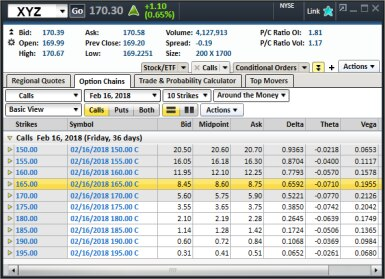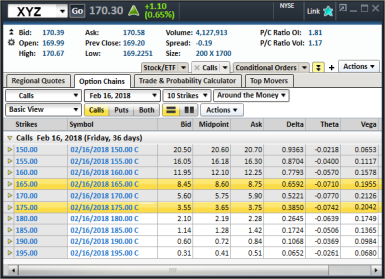Learning Center | April 12, 2018

# Option Strategy Spotlight: Long Call vs. Bull Call Spread

If you are a long option trader you are likely familiar with one of the biggest drawbacks of this strategy which is the impact of time decay. Once you purchase a long call or put you can expect that your option is going to lose a little bit of value every day until expiration, all other things being equal. An estimate of how much might be lost is expressed in the “Greek” measure known as Theta.

One way you can help offset the impact of time decay on a long option is by simultaneously selling another option against your initial position to form what is known as an option spread. There are other benefits that spreads can offer but like all option strategies there are also some trade-offs. In this article I’d like to compare a long call with a vertical bull call spread in order to help illustrate some of those benefits and risks.

#### Strategy comparison using an example

I find that the best way to help establish the grounds for a meaningful comparison is through the use of an example. Let’s assume that there are two traders who are equally bullish on XYZ in the near-term and intend to use options to potentially capitalize on that bullish forecast.

Trader #1 decides to purchase a long call while Trader #2 decides to establish a bull call spread. Let’s start by evaluating Trader #1’s long call strategy using some common strategy attributes and options Greeks, such as Delta, Theta and Vega. Then we will perform the same assessment on Trader #2’s bull call spread. Finally, we will put these two strategies side by side and review their respective benefits and trade-offs.

Long call example

To start, let’s assume that XYZ is trading for \$170.30 and trader #1 decides to purchase the 165.00 call that expires in 36 days (read more here about comparing strikes on long calls). With the help of some Greeks from the option chain below, let’s assess what this implies about this particular contract.Source: StreetSmart Edge®

XYZ 2/16/2018 165.00 C Summary

 Cost Break-Even Price Delta Theta Vega Maximum Gain 165.00 Call \$875.00 \$173.75 0.6592 -0.071 0.1955 Unlimited
• Cost: As you can see from the image above the 165.00 call has an ask price of \$8.75 so the total cost, taking the 100 mulitplier into account, is \$875.00, excluding commissions. The cost (as with any long options strategy) is also the maximum potential loss.
• Break-even price: The break-even price is calucalted by adding the cost of the 165.00 call to the strike price, so 165.00 + \$8.75 yields a break-even price of \$173.75. By purchasing this call trader #1 is essentially indicating that he or she believes that XYZ will close above the break-even price of \$173.75 at expiration (let’s assume this trader does not intend to  close out the position prior to expiration).
• Delta: The 0.6592 Delta has two implications – this particular call has an approximate 65% chance of closing in the money at expiration, and initially you can expect the value of this contract to increase/decrease by about \$0.6592 for a corresponding \$1.00 move higher/lower (respectively) in the price of XYZ. Delta is a dynamic number which changes when any of the other option pricing variables change.
• Theta: A Theta of -0.0710 suggests that initially the value of this contract is expected to lose roughly \$0.07 per day until expiration, all other variables held constant. Like Delta, and all of the Greeks for that matter, Theta is also a dynamic number which changes when any of the option pricing variables change.
• Vega: A Vega of 0.1955 tells you how much you can initially expect the value of the contract to change in reaction to a 1% change in the implied volatility of XYZ. For example, if the implied volatility on XYZ decreases 1% you can expect the value of this contract to initially lose \$0.1955.
• Maximum gain: Similar to a long stock position the price of XYZ really doesn’t have a theoretical limit to how high it can rise and therefore neither does the long call(at least up until expiration).

Now let’s turn to trader #2 who also decides to buy the 165.00 call which expires in 36 days but also decides to simultaneously sell the 175.00 call which expires at the same time, thereby establishing a 165.00/175.00 vertical bull call spread.Source: StreetSmart Edge®

XYZ 2/16/2018 165.00 C/175.00 C Summary

 Cost Break-Even Price Delta Theta Vega Maximum Gain 165.00 Call \$875.00 \$173.75 0.6592 -0.071 0.1955 175.00 Call -\$355.00 -0.3850 +0.0742 -0.2042 Combined Spread \$520.00 \$170.20 0.2742 +0.0032 -0.0087 \$480.00
• Cost: As we previously established the cost of the 165.00 call is \$8.75 so when taking into account the \$3.55 received from selling the 175.00 call the total cost of the spread is \$5.20, or \$520.00, when taking the 100 multiplier into account (excluding commissions). The \$520.00 cost of this spread represents the maxmimum potential loss and occurs if XYZ closes at or below \$165.00 at expiration.
• Break-even price: The break-even  price for this spread is calculated by adding the \$5.20 combined cost of the spread to the lower strike price of 165.00 which gives us a price of \$170.20 at expiration.
• Delta: The combined Delta for this bull call spread is calculated by subtracting the 0.385 Delta of the 175.00 call from the 0.6592 Delta of the 165.00 call. The Delta of the 175.00 call is subtracted from the 165.00 call since the contract is being sold and the combined spread Delta of 0.2742 indicates that the value of the overall spread will initially increase or decrease by about \$0.2742 with a corresponding \$1.00 move (higher or lower) in the price of XYZ.
• Theta: Since the 175.00 call is sold the Theta is positive since a short call gains value with the passage of time. Similar to Delta, this +0.0742 Theta is then combined with the -0.071 Theta of the 165.00 call, which gives us a positive 0.0032 Theta for the overall spread. While this suggests that the value of the spread will initially gain a little bit of value with the passage of time, keep in mind the Theta of each contract is subject to change with the passage of time, or when any of the option pricing variables are altered.
• Vega: Similar to Delta and Theta, the Vega of 0.1955 on the 165.00 call is combined with the negative Vega of the short 175.00 call which gives us a Vega of -0.0087 for the combined spread. This low Vega suggests that volatility risk has essentially been taken out of the equation. If implied volatility rises or falls significantly, the gain or loss from the long contract (i.e. the 165.00 call) is nearly completely offset by the corresponding gain or loss from the short contract (i.e. the 175.00 call).
• Maximum gain: The maximum gain of this bull call spread equals the distance between the two strikes, or \$10.00, minus the cost of the combined spread, or \$5.20. Therefore, the maximum gain is \$10.00-\$5.20 =\$4.80 or \$480.00, when taking the 100 multipler into consideration (excluding commissions). The maximum gain is achieved if XYZ closes at or above \$175.00 at expiration.

Now let’s compare these two strategies to get a better sense of their respective benefits and drawbacks.

Long call vs. bull call spread• Cost: Lower overall cost is a primary driver of establishing a spread and the bull call spread in this example costs about 40% less than the long call. Advantage: bull call spread
• Break-even price: In order for the long call to break-even the price of the underlying needs to increase by \$3.45 (\$173.75 - \$170.30) in 36 days. Conversely, at a current price of \$170.30, XYZ can go mainly sideways for the next 36 days and the bull call spread will still manage a small gain since the break-even price is \$170.20. Additionally, if you think of a trade strictly in terms of the “probability of making a profit”, and consider all of the possible XYZ closing prices at expiration, then the nod goes to the 165.00/175.00 bull call spread in this category. Advantage: bull call spread
• Delta: The disparity between the Delta’s of these two strategies highlights one of the biggest drawbacks of a spread trade: lower relative profit with a beneficial move in the price of the underlying. Both traders are bullish on XYZ but trader #1 will see the value of his call increase by about \$0.65 with a \$1.00 increase in XYZ while trader #2 will only see an increase of about \$0.27. As expiration approaches the combined Delta on the spread is likley to move closer to parity with the 165.00 call, but bull call spread traders need to be aware of this trade-off: the increase in value from the long option is going to be partially offset by the increase in value from the short option when you get a beneifical move in the price of the underlying. Advantage: long call
• Theta: Time decay is one of the biggest profit eliminators for long option traders and as you can see in the table above the 165.00 long call is initially set to lose approximately \$0.07, or nealy 1%, per day due to time decay. Conversely, you can see that not only has time decay been eliminated on the 165.00/175.00 bull call spread but there will actually be an initial gain after one day elapses (all other variables held constant). Advantage: bull call spread
• Vega: The value of the 165.00 long call stands to gain \$0.1955 with a 1% increase in implied volatility but lose \$0.1955 with a 1% drop in implied volatility. Therefore, you can see that there is “volatility risk” to the long call strategy. However, with a Vega of -0.0087 you can see that the 165.00/175.00 bull call spread has essentially eliminated volatility risk. Some might consider taking the volatility risk out of the equation a benefit of spread trading but since the benefit is not necessarily clear-cut we will mark this category as neutral. No advantage to either long call or bull call spread.
• Maximum gain: The potential for significant profits is probably one of the most alluring factors for long option traders. As you can see from above, the 165.00 long call offers (theoretical) unlimited upside while the 165.00/175.00 bull call spread can only achieve a \$480.00 profit at best. However, the maximum gain of \$480.00 profit on the bull call spread represents a 92% profit when compared to the \$520 cost (excluding commissions). Advantage: long call

#### Bottom line

The intent of this article is not to necessarily persuade you to select one strategy over another, it is meant to help shed light on the benefits and trade-offs to assist you when deciding which strategy to initiate. If you are comfortable with the risk and you are exceptionally bullish on a stock then you might prefer a long call strategy over a bull call spread since it offers more profit potential.

Having said that, even if you are bullish on a stock, it may not make the desired move within the needed timeframe in order to achieve a profit, so you might consider a bull call spread since it substantially reduces the time decay component. Regardless of how you proceed I hope this article has helped provide some insight into how these two strategies match-up. Look for additional bullish and bearish strategy comparisons in upcoming Option Strategy Spotlight articles.